# US5537259A - Zoom lens - Google Patents

## Info

Publication number
US5537259A
US5537259A US08/426,171 US42617195A US5537259A US 5537259 A US5537259 A US 5537259A US 42617195 A US42617195 A US 42617195A US 5537259 A US5537259 A US 5537259A
Authority
US
United States
Prior art keywords
lens
sub
zoom
refracting power
numerical
Prior art date
Legal status (The legal status is an assumption and is not a legal conclusion. Google has not performed a legal analysis and makes no representation as to the accuracy of the status listed.)
Application number
US08/426,171
Inventor
Hideki Ogawa
Current Assignee (The listed assignees may be inaccurate. Google has not performed a legal analysis and makes no representation or warranty as to the accuracy of the list.)
Canon Inc
Original Assignee
Canon Inc
Priority date (The priority date is an assumption and is not a legal conclusion. Google has not performed a legal analysis and makes no representation as to the accuracy of the date listed.)
Filing date
Publication date
Priority to JP6-088491 priority Critical
Priority to JP08849194A priority patent/JP3397440B2/en
Application filed by Canon Inc filed Critical Canon Inc
Assigned to CANON KABUSHIKI KAISHA reassignment CANON KABUSHIKI KAISHA ASSIGNMENT OF ASSIGNORS INTEREST (SEE DOCUMENT FOR DETAILS). Assignors: OGAWA, HIDEKI
Application granted granted Critical
Publication of US5537259A publication Critical patent/US5537259A/en
Anticipated expiration legal-status Critical
Expired - Lifetime legal-status Critical Current

• 230000005499 meniscus Effects 0.000 claims description 9
• 230000003287 optical Effects 0.000 abstract description 4
• 230000004075 alteration Effects 0.000 description 47
• 230000003247 decreasing Effects 0.000 description 6
• 239000000562 conjugates Substances 0.000 description 4
• 239000011521 glasses Substances 0.000 description 4
• 230000000694 effects Effects 0.000 description 2
• 230000004048 modification Effects 0.000 description 2
• 238000006011 modification reactions Methods 0.000 description 2
• 241000226585 Antennaria plantaginifolia Species 0.000 description 1
• 206010010071 Coma Diseases 0.000 description 1
• 239000003570 air Substances 0.000 description 1
• 230000002547 anomalous Effects 0.000 description 1
• 201000009310 astigmatism Diseases 0.000 description 1
• 239000006185 dispersions Substances 0.000 description 1
• 230000005484 gravity Effects 0.000 description 1
• 239000010950 nickel Substances 0.000 description 1
• 230000011514 reflex Effects 0.000 description 1
• 230000001629 suppression Effects 0.000 description 1

## Images

••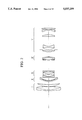•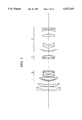•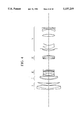•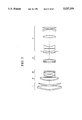•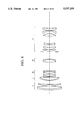•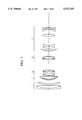•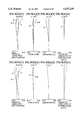•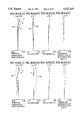•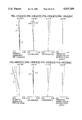••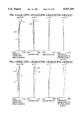•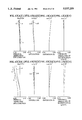•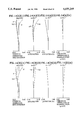•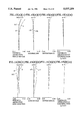•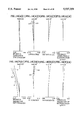••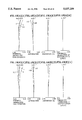•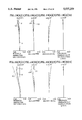•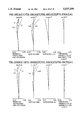•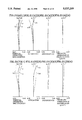•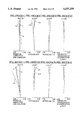•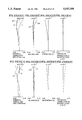•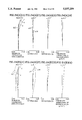•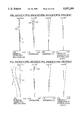## Classifications

• GPHYSICS
• G02OPTICS
• G02BOPTICAL ELEMENTS, SYSTEMS, OR APPARATUS
• G02B15/00Optical objectives with means for varying the magnification
• G02B15/14Optical objectives with means for varying the magnification by axial movement of one or more lenses or groups of lenses relative to the image plane for continuously varying the equivalent focal length of the objective
• G02B15/16Optical objectives with means for varying the magnification by axial movement of one or more lenses or groups of lenses relative to the image plane for continuously varying the equivalent focal length of the objective with interdependent non-linearly related movements between one lens or lens group, and another lens or lens group
• G02B15/163Optical objectives with means for varying the magnification by axial movement of one or more lenses or groups of lenses relative to the image plane for continuously varying the equivalent focal length of the objective with interdependent non-linearly related movements between one lens or lens group, and another lens or lens group having a first movable lens or lens group and a second movable lens or lens group, both in front of a fixed lens or lens group
• G02B15/167Optical objectives with means for varying the magnification by axial movement of one or more lenses or groups of lenses relative to the image plane for continuously varying the equivalent focal length of the objective with interdependent non-linearly related movements between one lens or lens group, and another lens or lens group having a first movable lens or lens group and a second movable lens or lens group, both in front of a fixed lens or lens group having an additional fixed front lens or group of lenses
• G02B15/173Optical objectives with means for varying the magnification by axial movement of one or more lenses or groups of lenses relative to the image plane for continuously varying the equivalent focal length of the objective with interdependent non-linearly related movements between one lens or lens group, and another lens or lens group having a first movable lens or lens group and a second movable lens or lens group, both in front of a fixed lens or lens group having an additional fixed front lens or group of lenses arranged +-+

## Abstract

A zoom lens includes at least a first lens group having a positive refracting power, a second lens group having a positive refracting power, and a third lens group having a negative refracting power in this order from the object side. Such zoom lens performs zooming by varying the distance between respective lens groups. Zooming from the wide angle end to the telephoto end is performed by moving the second and third lens groups toward the image plane side at different speeds, and focusing is performed by moving the second lens group. Various optical conditions are satisfied in the zoom lens.

## Description

BACKGROUND OF THE INVENTION
1. Field of the Invention
The present invention relates to a zoom lens which is suitable for a 35 mm single-lens reflex camera and, more particularly, to a zoom lens suitable for an autofocus camera in which aberrations are satisfactorily corrected although the zoom lens has a focal length at the wide angle end of about 70 mm, a zoom ratio of about 3:1, and a relatively large aperture of an F number of about 2.8.
2. Description of the Related Art
In recent years, in an autofocus camera which is increasingly the subject of development, not only is the performance of accurate focusing important, but also the attainment of rapid focus speed. The focus speed is determined mainly by power supplied to a focus motor, the efficiency of the power focus motor, the load of a lens barrel, the weight and extension of the focus lens, the computation processing speed of the autofocus, and the like. Of these factors, the factors which influence focus speed the most are the weight of the focus lens and the extension thereof. It is therefore important to achieve an optical design in which the influence of the weight and extension factors is limited to be small.
A zoom lens, having a focal length at the wide angle end of about 70 mm, a zoom ratio of about 3:1, and an F number of about 2.8 (constant during zooming), is generally formed of a first lens group having a positive refracting power, a second lens group having a negative refracting power, a third lens group having a positive refracting power, and a fourth lens group having a positive refracting power. Such a lens is well known and afocal zooming is performed by moving the second and third groups during zooming while the first and fourth groups are fixed and focusing is performed by the first lens group (front lens focusing) or a part of the first lens group (front lens inner focusing). An example of front lens focusing is proposed in, for example, Japanese Patent Laid-Open Nos. 62-108218, 64-39542, and 63-58324. Although the front lens has a relatively small aberration, the weight of the front lens is fairly heavy when it has a large aperture, for example, when the F number is F2.8. When the closest focusing distance is set at a relatively short distance, for example, about 1.5 m, the extension amount is increased relatively. Therefore, it cannot be said that the zoom lens is suitable for an autofocus camera. When the power of the front lens is increased in order to decrease the extension amount, the spherical aberration, in particular, at a near distance on the long focal point side, will become great and be undercorrected, which is problematic.
An example of front lens inner focusing is disclosed in, for example, Japanese Examined Patent No. 61-053696. Since focusing is performed by a lens group disposed relatively on the image side of the front lens, it is possible to decrease the lens diameter to some extent, and as a result, lessen the weight of the focus lens to some extent. There are advantages to this type of system even if the refracting power is increased to decrease the extension amount. For example, little aberration will occur due to the floating effect with the lens group of the front lens on the object side. Despite the advantages, such a system is nonetheless not suitable for an autofocus camera in terms of its weight.
Further examples in which the weight of the focus lens is reduced are disclosed in, for example, Japanese Examined Patent Nos. 1-25044 and 1-25043. These examples are designed to focus using a part of the fixed fourth lens group (relay); however, there are problems associated with this system, for example, aberrations, such as a spherical aberration or an astigmatism, will be large though the weight of the focus lens is fairly small.
SUMMARY OF THE INVENTION
It is an object of the present invention to provide a high-performance zoom lens which is suitable for an autofocus camera, in which the weight of the focus lens and the extension amount thereof are reduced while utilizing the advantages of the aberration characteristics of front lens inner focusing in order to eliminate the above-described problems of the prior art.
To achieve the above-described object, according to the present invention, there is provided a zoom lens comprising: at least a first lens group having a positive refracting power, a second lens group having a positive refracting power, and a third lens group having a negative refracting power in this order from the object side, which performs zooming by varying the distance between respective lens groups, wherein zooming from the wide angle end to the telephoto end is performed by moving the second and third lens groups toward the image side at different speeds and focusing is performed by the second lens group, and the condition described below is satisfied:
`0,025<m.sub.2 /f.sub.T <0.135 (m.sub.2 >0)                 (1)`
where fT is the focal distance at the telephoto end of the entire system, and m2 is the amount of zoom movement of the second lens group from the wide angle end to the telephoto end.
The above and further objects and novel features of the invention will more fully appear from the following detailed description when read in connection with the accompanying drawings.
BRIEF DESCRIPTION OF THE DRAWINGS
FIGS. 1(a) and 1(b) show the paraxial refracting power arrangement of a zoom lens in accordance with the present invention;
FIG. 2 is a sectional view of lenses in accordance with a first numerical embodiment of the zoom lens in accordance with the present invention;
FIG. 3 is a sectional view of lenses in accordance with a second numerical embodiment of the zoom lens in accordance with the present invention;
FIG. 4 is a sectional view of lenses in accordance with a third numerical embodiment of the zoom lens in accordance with the present invention;
FIG. 5 is a sectional view of lenses in accordance with a fourth numerical embodiment of the zoom lens in accordance with the present invention;
FIG. 6 is a sectional view of lenses in accordance with a fifth numerical embodiment of the zoom lens in accordance with the present invention;
FIG. 7 is a sectional view of lenses in accordance with a sixth numerical embodiment of the zoom lens in accordance with the present invention;
FIGS. 8(A) and 8(B) show various aberrations at the wide angle end in accordance with the first numerical embodiment of the zoom lens of the present invention;
FIGS. 9(A) and 9(B) show various aberrations at the intermediate region in accordance with the first numerical embodiment of the zoom lens of the present invention;
FIGS. 10(A) and 10(B) show various aberrations at the telephoto end in accordance with the first numerical embodiment of the zoom lens of the present invention;
FIGS. 11(A) and 11(B) show various aberrations at the wide angle end in accordance with the second numerical embodiment of the zoom lens of the present invention;
FIGS. 12(A) and 12(B) show various aberrations at the intermediate region in accordance with the second numerical embodiment of the zoom lens of the present invention;
FIGS. 13(A) and 13(B) show various aberrations at the telephoto end in accordance with the second numerical embodiment of the zoom lens of the present invention;
FIGS. 14(A) and 14(B) show various aberrations at the wide angle end in accordance with the third numerical embodiment of the zoom lens of the present invention;
FIGS. 15(A) and 15(B) show various aberrations at the intermediate region in accordance with the third numerical embodiment of the zoom lens of the present invention;
FIGS. 16(A) and 16(B) show various aberrations at the telephoto end in accordance with the third numerical embodiment of the zoom lens of the present invention;
FIGS. 17(A) and 17(B) show various aberrations at the wide angle end in accordance with the fourth numerical embodiment of the zoom lens of the present invention;
FIGS. 18(A) and 18(B) show various aberrations at the intermediate region in accordance with the fourth numerical embodiment of the zoom lens of the present invention;
FIGS. 19(A) and 19(B) show various aberrations at the telephoto end in accordance with the fourth numerical embodiment of the zoom lens of the present invention;
FIGS. 20(A) and 20(B) show various aberrations at the wide angle end in accordance with the fifth numerical embodiment of the zoom lens of the present invention;
FIGS. 21(A) and 21(B) show various aberrations at the intermediate region in accordance with the fifth numerical embodiment of the zoom lens of the present invention;
FIGS. 22(A) and 22(B) show various aberrations at the telephoto end in accordance with the fifth numerical embodiment of the zoom lens of the present invention;
FIGS. 23(A) and 23(B) show various aberrations at the wide angle end in accordance with the sixth numerical embodiment of the zoom lens of the present invention;
FIGS. 24(A) and 24(B) show various aberrations at the intermediate region in accordance with the sixth numerical embodiment of the zoom lens of the present invention; and
FIGS. 25(A) and 25(B) show various aberrations at the telephoto end in accordance with the sixth numerical embodiment of the zoom lens of the present invention.
DESCRIPTION OF THE PREFERRED EMBODIMENTS
Preferred embodiments of the present invention will be described below.
FIGS. 1(a) and 1(b) show the paraxial refracting power arrangement of a zoom lens in accordance with the present invention. In particular, FIG. 1(a) shows the state in which the axial light beam is passed at the short focal point end; FIG. 1(b) shows the state in which the paraxial light beam is passed at the long focal point end. Reference characters I to V denote the first to fifth lens groups, respectively, from the object side to the image side. Zooming is increased by moving the third lens group having a negative refracting power toward the image side, while the first lens group having a positive refracting power and the fifth lens group having a positive refracting power are fixed. The variation of the image point caused by zooming is corrected primarily by moving the fourth lens group having a positive refracting power. During this operation, the second lens group is moved in such a manner as to move away from the first lens group, i.e., toward the image plane, and to the long focal length side of the range in which the conditional equation (1) described above is satisfied.
When a change of the paraxial light beam to the first lens group is seen during zooming, the change is low on the short focal-point side and conversely high on the long focal-point side, and the paraxial light beam converges as a result of the positive refracting power of the first lens group. Thus, the second lens group is moved as described above in order to decrease the outer diameter of the lenses of the second lens group determined by the incidence height of the paraxial light beam on the long focal point side.
FIGS. 2 to 7 are sectional views of lenses shown in numerical embodiments which will be described later. Reference characters I to V denote first to fifth lens groups, respectively.
Conditional equation (1) is concerned with the amount of movement of zooming at the long focal point end with the short focal point side end of the second lens group being a reference, and defines a condition for reducing the weight of the lenses while satisfactorily suppressing the aberration. If the amount of movement is increased beyond the upper limit of conditional equation (1), the lens weight is reduced because the outer diameter of the lenses is decreased; however, a reduction effect is increased during zooming because of the positive refracting power of the second lens group. Thus, both the negative refracting power of the third lens group and the positive refracting power of the fourth lens group for the purpose of obtaining a desired zoom ratio are increased, and therefore, even if the number of lenses of the third and fourth lens groups is increased, variations due to zooming, such as spherical aberration or coma aberration, cannot be removed. In addition, at the long focal-point side, the distortion aberration will greatly vary, and pin-cushion distortion will become conspicuous, which is undesirable.
If, conversely, the amount of movement is decreased beyond the lower limit of conditional equation (1), the refracting powers of the third and fourth lens groups are decreased. This is advantageous as regards aberration correction; however, as the outer diameter of the lenses is increased, reducing the weight of the lenses becomes difficult.
Although the object of the present invention can be sufficiently achieved without changing the numerical range of conditional equation (1), more preferably, the range should be the following numerical range:
`0.09<m.sub.2 /f.sub.T <0.13                                (1')`
Still more preferably, if the focal lengths of the first and second lens groups are denoted as f1 and f2, respectively, and the following condition is satisfied:
`0.75<f.sub.1 /f.sub.2 <1.50                                (2)`
it is easy to give optical performance for practical use.
Conditional equation (2) is concerned with the ratio of the refracting power of the first lens group to that of the second lens group. Conditional equation (2) satisfies conditional equation (1), and also regulates the amount of focus extension of the second lens group while correcting aberration satisfactorily.
If the refracting power of the first lens group is increased and if the refracting power of the second lens group is decreased beyond the lower limit of conditional equation (2), various aberrations which occur in the first lens group increase, thus it is difficult to cancel the aberrations by the second and subsequent lens groups. Further, not only is the amount of extension of the second lens group during focusing increased, but also the lens diameter is increased as the amount of extension is increased, and the weight of the lenses is increased, which are undesirable.
If, conversely, mainly the refracting power of the second lens group is increased beyond the upper limit of conditional equation (2), the amount of extension of the lenses is decreased; however, the spherical aberration and the chromatic aberration (both of the axial color and the chromatic aberration of magnification) will vary greatly, in particular, during focusing, and it is difficult to correct these aberrations. Further, the refracting powers of the third and fourth lens groups for obtaining a desired zoom ratio are increased due to the influence of the positive refracting power of the second lens group, and it is difficult to correct the aberration variations caused by zooming, as described in conditional equation (1) described above, which is undesirable.
Although the object of the present invention can be achieved sufficiently without changing the numerical range of conditional equation (2), more preferably, the numerical range should be the following range:
`0.8<f.sub.1 /f.sub.2 <1.45                                 (2')`
Still more preferably, the following condition should be satisfied:
`0.92<f.sub.1 /f.sub.2 <1.4                                 (2")`
It is more preferable that the value of the ratio of f2 to fT be in the numerical range below as a condition for optimizing the aberration correction and the amount of extension:
`0.65<f.sub.2 /f.sub.T <1.50                                (3)`
Although it is possible to sufficiently balance the aberration correction and the amount of extension in the numerical range of equation (3), more preferably, the range should be the following range:
`0.7<f.sub.2 /f.sub.T <1.2                                  (3')`
The zoom arrangement is desirably formed in such a way that a fixed fifth lens group having a positive refracting power is provided on the image plane side of the fourth lens group, and an afocal zooming system is formed by the first to fourth lens groups, as in the embodiment of the present invention.
The first lens group is formed of a meniscus negative lens having a greatly concaved surface on the image side, a double convex lens and a meniscus positive lens having a greatly convexed surface on the object side in this order from the object side. The second lens group is preferably formed of a meniscus negative lens having a concave surface on the image side and a positive lens whose curvature of the lens surface on the object side is greater than that of the lens surface on the image side. The third lens group is formed of a negative lens having a greatly concaved surface on the image side and a cemented lens comprising a negative and a positive lens, and a meniscus negative lens having a concave surface on the object side. The fourth lens group is formed of at least one positive lens and a meniscus negative lens having a concave surface on the object side. The fifth lens group is formed of a positive lens, a positive lens having a greatly convexed surface on the object side, a negative lens having a greatly concave surface on the image side, a positive lens, a meniscus negative lens having a concave surface on the object side, and a positive lens. Thus, excellent optical performance is maintained.
Use of a glass member with an anomalous dispersion ratio having a refractive index of about 1.5 and an abbe number of about 80 in at least one of the two positive lenses of the first lens group makes it possible to satisfactorily correct chromatic aberration. A glass member having a relatively small specific gravity and a refractive index of 1.65 or less may preferably be used as the positive lens of the second lens group, and a glass member having an abbe number of 40 or more may preferably be used by taking the correction of chromatic aberration into consideration.
Following conditional equation (3) described earlier, it is more preferable that any of conditional equations (4), (5) and (6) described below be satisfied. If the focal length of the third lens group is denoted as f3, and if the value of the ratio of f3 to fT is set within the range:
`0.12<|f.sub.3 |/f.sub.T <0.17            (4)`
then, various conditions, such as a zoom ratio, a total length of the lenses, or a back focus, are satisfied, and it is easy to correct aberrations satisfactorily.
If the focal length of the fourth lens group is denoted as f4, and if the value of the ratio of f4 to fT is set within the following range:
`0.35<f.sub.4 /f.sub.T <0.7                                 (5)`
then, various conditions, such as a zoom ratio, a total length of the lenses, or a back focus, are satisfied and it is easy to correct aberrations satisfactorily.
If the focal length of the fifth lens group is denoted as f5, and if the value of the ratio of f5 to fT is set within the following range:
`0.3<f.sub.5 /f.sub.T <0.7                                  (6)`
then, various conditions, such as a zoom ratio, a total length of the lenses, or a back focus, are satisfied, and it is easy to correct aberrations satisfactorily.
Next, numerical embodiments of the present invention will be described. In the numerical embodiments, reference character Ri denotes the curvature radius of the i-th lens surface sequentially from the first conjugate point; reference character Di denotes the thickness of the i-th lens and an air gap from the first conjugate point; reference characters Ni and vi denote a refractive index of glass of the i-th lens from the conjugate point and its abbe number, respectively, sequentially from the first conjugate point.
The relation between each of the above-described conditional equations and the numerical values in the numerical embodiments is shown in Table 1.
In the aberration figures, (A) shows a figure with respect to an object at infinity, and (B) shows a figure when the zoom lens is focused on an object at a distance of 1.5 m.
`______________________________________First Numerical Embodiment     fno = 1:2.923              f = 72.10˜194.01______________________________________r1 = 311.919       d1 = 2.80  n1 = 1.74950                              ν1 = 35.0r2 = 118.630       d2 = 0.42r3 = 128.135       d3 = 8.68  n2 = 1.49700                              ν2 = 81.6r4 = -263.474       d4 = 0.10r5 = 79.501 d5 = 5.72  n3 = 1.49700                              ν3 = 81.6r6 = 203.191       d6 =       VARIABLEr7 = 54.391 d7 = 2.20  n4 = 1.84666                              ν4 = 23.8r8 = 45.859 d8 = 1.13r9 = 51.927 d9 = 8.55  n5 = 1.48749                              ν5 = 70.2r10 = 5099.296       d10 =       VARIABLEr11 = -488.600       d11 = 1.40 n6 = 1.80400                              ν6 = 46.6r12 = 35.390       d12 = 5.88r13 = -78.125       d13 = 1.40 n7 = 1.48749                              ν7 = 70.2r14 = 38.137       d14 = 4.97 n8 = 1.84666                              ν8 = 23.9r15 = 417.478       d15 = 2.65r16 = -66.802       d16 = 1.40 n9 = 1.72916                              ν9 = 54.7r17 = -3362.971       d17 =       VARIABLEr18 = 247.120       d18 = 3.49 n10 = 1.69680                              ν10 = 55.5r19 = -99.902       d19 = 0.15r20 = -189.999       d20 = 4.77 n11 = 1.49700                              ν11 = 81.6r21 = -40.553       d21 = 1.45 n12 = 1.83400                              ν12 = 37.2r22 = -76.277       d22 =       VARIABLEr23 = 58.421       d23 = 3.53 n13 = 1.80400                              ν13 = 46.6r24 = 133.262       d24 = 3.00r25 = (ABER-       d25 = 0.24RATION)r26 = 34.132       d26 = 6.35 n14 = 1.49700                              ν14 = 81.6r27 = 2256.763       d27 = 3.72 n15 = 1.62004                              ν15 = 36.3r28 = 31.519       d28 = 28.17r29 = 132.947       d29 = 5.90 n16 = 1.51742                              ν16 = 52.4r30 = -77.546       d30 = 13.94r31 = -39.485       d31 = 1.80 n17 = 1.83400                              ν17 = 37.2r32 = -95.683       d32 = 0.15r33 = 147.644       d33 = 3.62 n18 = 1.74320                              ν18 = 49.3r34 = -205.762______________________________________VARIABLE    FOCAL LENGTHDISTANCE    72.10       135.00  194.01______________________________________d6          8.78        26.15   32.85d10         1.64        12.89   17.23d17         30.32       15.72   1.32d22         14.70       0.69    4.05______________________________________`
`______________________________________Second Numerical Embodiment     fno = 1:2.924              f = 72.10˜93.90______________________________________r1 = 225.542       d1 = 2.5   n1 = 1.74950                              ν1 = 35.0r2 = 104.642       d2 = 0.28r3 = 108.610       d3 = 9.27  n2 = 1.49700                              ν2 = 81.6r4 = -265.354       d4 = 0.10r5 = 75.092 d5 = 4.70  n3 = 1.49700                              ν3 = 81.6r6 = 140.045       d6 =       VARIABLEr7 = 59.544 d7 = 1.60  n4 = 1.84666                              ν4 = 23.8r8 = 49.090 d8 = 0.99r9 = 54.762 d9 = 7.79  n5 = 1.52542                              ν5 = 64.6r10 = 1743.971       d10 =       VARIABLEr11 = -530.206       d11 = 1.40 n6 = 1.83481                              ν6 = 42.7r12 = 37.558       d12 = 5.68r13 = -79.170       d13 = 1.40 n7 = 1.48749                              ν7 = 70.2r14 = 40.477       d14 = 5.04 n8 = 1.84666                              ν8 = 23.9r15 = 2687.975       d15 = 2.31r16 = -69.555       d16 = 1.40 n9 = 1.71300                              ν9 = 53.8r17 = -2323.188       d17 =       VARIABLEr18 = 221.017       d18 = 3.19 n10 = 1.60311                              ν10 = 60.7r19 = -138.222       d19 = 0.15r20 = 1868.115       d20 = 5.61 n11 = 1.49700                              ν11 = 81.6r21 = -44.515       d21 = 1.40 n12 = 1.83400                              ν12 = 37.2r22 = -84.287       d22 =       VARIABLEr23 = 50.371       d23 = 3.37 n13 = 1.78800                              ν13 = 47.4r24 = 96.720       d24 = 3.40r25 = (ABBER-       d25 = 7.83RATION)r26 = 35.719       d26 = 6.29 n14 = 1.49700                              ν14 = 81.6r27 = 364.987       d27 = 0.18r28 = 426.065       d28 = 4.23 n15 = 1.64769                              ν15 = 33.8r29 = 30.169       d29 = 18.38r30 = 564.115       d30 = 3.70 n16 = 1.62374                              ν16 = 47.1r31 = -75.863       d31 = 14.24r32 = -41.096       d32 = 1.80 n17 = 1.83400                              ν17 = 37.2r33 = -79.119       d33 = 0.15r34 = 88.271       d34 = 3.22 n18 = 1.83481                              ν18 = 42.7r35 = 631.452______________________________________VARIABLE    FOCAL LENGTHDISTANCE    72.10       135.00  193.90______________________________________d6          8.62        26.51   33.11d10         1.50        12.82   17.00d17         36.70       18.29   1.03d22         12.22       1.42    7.90______________________________________`
`______________________________________Third Numerical Embodiment     fno = 1:2.923              f = 72.10˜194.01______________________________________r1 = 276.573       d1 = 2.80  n1 = 1.74950                              ν1 = 35.0r2 = 113.188       d2 = 0.31r3 = 118.700       d3 = 9.18  n2 = 1.49700                              ν2 = 81.6r4 = -248.684       d4 = 0.10r5 = 79.217 d5 = 5.61  n3 = 1.49700                              ν3 = 81.6r6 = 189.619       d6 =       VARIABLEr7 = 58.290 d7 = 2.20  n4 = 1.84666                              ν4 = 23.8r8 = 48.706 d8 = 0.89r9 = 53.632 d9 = 8.16  n5 = 1.48749                              ν5 = 70.2r10 = 1473.054       d10 =       VARIABLEr11 = -456.797       d11 = 1.40 n6 = 1.80400                              ν6 = 46.6r12 = 35.948       d12 = 5.74r13 = -77.752       d13 = 1.40 n7 = 1.48749                              ν7 = 70.2r14 = 38.943       d14 = 4.96 n8 = 1.84666                              ν8 = 23.9r15 = 458.710       d15 = 2.51r16 = -68.075       d16 = 1.40 n9 = 1.72916                              ν9 = 54.7r17 = -1027.347       d17 =       VARIABLEr18 = 226.746       d18 = 3.51 n10 = 1.69680                              ν10 = 55.5r19 = -104.460       d19 = 0.15r20 = -255.232       d20 = 4.86 n11 = 1.49700                              ν11 = 81.6r21 = -42.121       d21 = 1.45 n12 = 1.83400                              ν12 = 37.2r22 = -84.183       d22 =       VARIABLEr23 = 56.799       d23 = 4.04 n13 = 1.80400                              ν13 = 46.6r24 = 117.472       d24 = 3.14r25 = (ABER-       d25 = 0.23RATION)r26 = 33.786       d26 = 6.02 n14 = 1.49700                              ν14 = 81.6r27 = 647.995       d27 = 5.02 n15 = 1.62004                              ν15 = 36.3r28 = 30.401       d28 = 26.69r29 = 140.183       d29 = 5.85 n16 = 1.51742                              ν16 = 52.4r30 = -75.171       d30 = 13.54r31 = -39.511       d31 = 1.80 n17 = 1.83400                              ν17 = 37.2r32 = -87.264       d32 = 0.15r33 = 110.673       d33 = 3.51 n18 = 1.74320                              ν18 = 49.3r34 = -540.841______________________________________VARIABLE    FOCAL LENGTHDISTANCE    72.10       135.00  194.01______________________________________d6          8.68        25.94   32.52d10         1.50        12.69   16.94d17         32.17       16.61   1.44d22         13.54       0.65    4.98______________________________________`
`______________________________________Fourth Numerical Embodiment     fno = :2.923              f = 72.10˜193.26______________________________________r1 = 110.141       d1 = 2.50  n1 = 1.84666                              ν1 = 23.8r2 = 87.208 d2 = 0.69r3 = 95.385 d3 = 8.75  n2 = 1.49700                              ν2 = 81.6r4 = -590.187       d4 = 0.10r5 = 82.563 d5 = 3.72  n3 = 1.49700                              ν3 = 81.6r6 = 127.384       d6 =       VARIABLEr7 = 71.160 d7 = 1.60  n4 = 1.84666                              ν4 = 23.8r8 = 56.304 d8 = 0.55r9 = 60.948 d9 = 6.52  n5 = 1.55232                              ν5 = 63.8r10 = 593.939       d10 =       VARIABLEr11 = -592.481       d11 = 1.40 n6 = 1.88300                              ν6 = 40.8r12 = 35.493       d12 = 5.60r13 = -65.813       d13 = 1.40 n7 = 1.49700                              ν7 = 81.6r14 = 38.705       d14 = 5.23 n8 = 1.84666                              ν8 = 23.8r15 = -796.747       d15 = 2.24r16 = -61.323       d16 = 1.40 n9 = 1.71300                              ν9 = 53.8r17 = -1138.043       d17 =       VARIABLEr18 = 199.355       d18 = 3.67 n10 = 1.63854                              ν10 = 55.4r19 = -103.069       d19 = 0.15r20 = -908.853       d20 = 5.79 n11 = 1.49700                              ν11 = 81.6r21 = -39.561       d21 = 1.40 n12 = 1.85026                              ν12 = 32.3r22 = -73.502       d22 =       VARIABLEr23 = 89.528       d23 = 2.91 n13 = 1.78300                              ν13 = 36.2r24 = 273.169       d24 = 2.00r25 = (ABER-       d25 = 0.50RATION)r26 = 41.342       d26 = 8.60 n14 = 1.49700                              ν14 = 81.6r27 = -657.295       d27 = 0.58r28 = -280.406       d28 = 5.30 n15 = 1.65446                              ν15 = 33.6r29 = 42.786       d29 = 25.54r30 = 155.211       d30 = 5.70 n16 = 1.61272                              ν16 = 58.8r31 = -66.167       d31 = 18.25r32 = -39.523       d32 = 1.80 n17 = 1.86403                              ν17 = 37.2r33 = -94.718       d33 = 0.42r34 = 96.052       d34 = 2.86 n18 = 1.86300                              ν18 = 41.5r35 = 363.586______________________________________VARIABLE    FOCAL LENGTHDISTANCE    72.10       135.00  193.26______________________________________d6          11.32       25.51   30.81d10         1.50        15.65   20.94d17         25.22       12.92   1.17d22         17.25       1.21    2.37______________________________________`
`______________________________________Fifth Numerical Embodiment     fno = 1:2.94              f = 72.10˜203.51______________________________________r1 = 171.950       d1 = 3.00  n1 = 1.80518                              ν1 = 25.4r2 = 115.156       d2 = 0.13r3 = 121.598       d3 = 7.97  n2 = 1.49700                              ν2 = 81.6r4 = -407.029       d4 = 0.10r5 = 99.019 d5 = 4.62  n3 = 1.49700                              ν3 = 81.6r6 = 216.142       d6 =       VARIABLEr7 = 84.581 d7 = 1.30  n4 = 1.84666                              ν4 = 23.8r8 = 59.833 d8 = 7.16  n5 = 1.62012                              ν5 = 49.5r9 = 456.702       d9 =       VARIABLEr10 = 1538.228       d10 = 1.40 n6 = 1.80400                              ν6 = 46.6r11 = 37.318       d11 = 4.99r12 = -131.265       d12 = 1.40 n7 = 1.58913                              ν7 = 61.2r13 = 35.604       d13 = 5.33 n8 = 1.84666                              ν8 = 23.9r14 = 455.335       d14 = 3.00r15 = -50.362       d15 = 1.40 n9 = 1.69680                              ν9 = 55.5r16 = -418.859       d16 =       VARIABLEr17 = 188.059       d17 = 7.62 n10 = 1.49700                              ν10 = 81.6r18 = -34.077       d18 = 1.40 n11 = 1.83400                              ν11 = 37.2r19 = -52.306       d19 =       VARIABLEr20 = (ABER-       d20 = 1.00RATION)r21 = 57.806       d21 = 4.65 n12 = 1.49700                              ν12 = 81.6r22 = -1836.788       d22 = 0.14r23 = 32.883       d23 = 7.26 n13 = 1.49700                              ν13 = 81.6r24 = 102.468       d24 = 7.85r25 = -371.499       d25 = 2.00 n14 = 1.60342                              ν14 = 38.0r26 = 36.388       d26 = 23.04r27 = 167.965       d27 = 6.96 n15 = 1.54814                              ν15 = 45.8r28 = -39.615       d28 = 6.98r29 = - 28.542       d29 = 1.80 n16 = 1.83400                              ν16 = 37.2r30 = -78.734       d30 = 0.42r31 = 61.694       d31 = 5.97 n17 = 1.77250                              ν17 = 49.6r32 = 244.286______________________________________VARIABLE    FOCAL LENGTHDISTANCE    72.10       135.00  203.51______________________________________d6          12.13       29.14   36.75d9          1.12        14.90   21.07d16         25.33       14.53   0.51d19         21.07       1.09    1.33______________________________________`
`______________________________________Sixth Numerical Embodiment     fno = 1:2.923              f = 72.10˜94.01______________________________________r1 = 355.855       d1 = 2.80  n1 = 1.74950                              ν1 = 35.0r2 = 121.211       d2 = 0.42r3 = 131.256       d3 = 8.62  n2 = 1.49700                              ν2 = 81.6r4 = -259.209       d4 = 0.10r5 = 80.584 d5 = 6.01  n3 = 1.49700                              ν3 = 81.6r6 = 234.800       d6 =       VARIABLEr7 = 51.450 d7 = 2.20  n4 = 1.84666                              ν4 = 23.8r8 = 43.769 d8 = 1.28r9 = 49.946 d9 = 8.87  n5 = 1.48749                              ν5 = 70.2r10 = 12148.909       d10 =       VARIABLEr11 = -600.368       d11 = 1.40 n6 = 1.80400                              ν6 = 46.6r12 = 34.801       d12 = 5.98r13 = -75.966       d13 = 1.40 n7 = 1.48749                              ν7 = 70.2r14 = 37.777       d14 = 4.97 n8 = 1.84666                              ν8 = 23.9r15 = 413.301       d15 = 2.64r16 = -66.400       d16 = 1.40 n9 = 1.72916                              ν9 = 54.7r17 = 3021.469       d17 =       VARIABLEr18 = 230.258       d18 = 3.51 n10 = 1.69680                              ν10 = 55.5r19 = -98.917       d19 = 0.15r20 = -172.378       d20 = 4.66 n11 = 1.49700                              ν11 = 81.6r21 = -40.226       d21 = 1.45 n12 = 1.83400                              ν12 = 37.2r22 = -76.185       d22 =       VARIABLEr23 = 57.653       d23 = 3.73 n13 = 1.80400                              ν13 = 46.6r24 = 128.671       d24 = 3.05r25 = (ABER-       d25 = 0.34RATION)r26 = 33.882       d26 = 6.26 n14 = 1.49700                              ν14 = 81.6r27 = 1455.342       d27 = 3.99 n15 = 1.62004                              ν15 = 36.3r28 = 31.129       d28 = 26.85r29 = 117.922       d29 = 5.91 n16 = 1.51742                              ν16 = 52.4r30 = -81.244       d30 = 14.02r31 = -38.692       d31 = 1.80 n17 = 1.83400                              ν17 = 37.2r32 = -102.301       d32 = 0.15r33 = 183.092       d33 = 3.91 n18 = 1.74320                              ν18 = 49.3r34 = -129.948______________________________________VARIABLE    FOCAL LENGTHDISTANCE    72.10       135.00  194.01______________________________________d6          8.69        26.10   32.84d10         1.57        12.05   16.10d17         30.32       15.57   0.97d22         13.86       0.71    4.52______________________________________`
`                                  TABLE 1__________________________________________________________________________FIRST        SECOND   THIRD    FOURTH   FIFTH    SIXTHEMBODIMENT   EMBODIMENT                 EMBODIMENT                          EMBODIMENT                                   EMBODIMENT                                            EMBODIMENT__________________________________________________________________________m.sub.2 /f.sub.T    0.124    0.126    0.123    0.101    0.121    0.124f.sub.1 /f.sub.2    1.150    1.112    1.015    0.821    0.862    1.225f.sub.2 /f.sub.T    0.781    0.806    0.848    1.014    0.995    0.731f.sub.3 /f.sub.T    -0.140   -0.154   -0.145   -0.138   -0.140   -0.137f.sub.4 /f.sub.T    0.531    0.538    0.536    0.456    0.565    0.533f.sub.5 /f.sub.T    0.483    0.542    0.495    0.495    0.414    0.476__________________________________________________________________________`
As has been described up to this point, by defining the movement of the second lens group so as to satisfy conditional equation (1) described earlier, the diameter of the second lens group can be decreased and focusing can be made speedier. In addition, by defining the focal lengths of the first and second lens groups so as to satisfy conditional equation (2), it is possible to provide an excellent zoom lens free from aberration.
Many different embodiments of the present invention may be constructed without departing from the spirit and scope of the present invention. It should be understood that the present invention is not limited to the specific embodiments described in this specification. To the contrary, the present invention is intended to cover various modifications and equivalent arrangements included within the spirit and scope of the claims. The following claims are to be accorded the broadest interpretation so as to encompass all such modifications, equivalent structures and functions.

## Claims (5)

What is claimed is:
1. A zoom lens comprising at least a first lens group having a positive refracting power, a second lens group having a positive refracting power, and a third lens group having a negative refracting power in this order from an object side to an image side, wherein zooming from a wide angle end to a telephoto end is performed by moving said second and third lens groups toward the image side at different speeds, focusing is performed by moving said second lens group, and the following condition is satisfied:
`0.025<m.sub.2 /f.sub.T <0.135 (m.sub.2 >0)`
where fT is the focal length at the telephoto end of the entire system, and m2 is the amount of zoom movement of said second lens group from the wide angle end to the telephoto end.
2. A zoom lens according to claim 1, wherein the focal lengths of said first and second lens groups are denoted as f1 and f2, respectively, and the following condition is satisfied:
`0.75<f.sub.1 /f.sub.2 <1.50.`
3. A zoom lens according to claim 1, further comprising a fourth lens group adjacent said third lens group having a positive refracting power, and a fifth lens group adjacent said fourth lens group having a positive refracting power, wherein an afocal zooming system is formed by said first to fourth lens groups, and said fifth lens group is fixed during zooming.
4. A zoom lens according to claim 3, wherein the following relation is satisfied:
`0.65<f.sub.2 /f.sub.T <1.50`
wherein f2 is the focal lenth of said second lens group.
5. A zoom lens according to claim 3, wherein said first lens group has a meniscus negative lens having a greatly concaved surface on the image side, a double convex lens, and a meniscus positive lens having a greatly convexed surface on the object side in this order from the object side toward the image side, and said second lens group has a meniscus negative lens having a concave surface on the image side and a positive lens whose curvature of a lens surface on the object side is greater than that of a lens surface on the image side.
US08/426,171 1994-04-26 1995-04-21 Zoom lens Expired - Lifetime US5537259A (en)

## Priority Applications (2)

Application Number Priority Date Filing Date Title
JP6-088491 1994-04-26
JP08849194A JP3397440B2 (en) 1994-04-26 1994-04-26 Zoom lens

## Publications (1)

Publication Number Publication Date
US5537259A true US5537259A (en) 1996-07-16

# Family

## Family Applications (1)

Application Number Title Priority Date Filing Date
US08/426,171 Expired - Lifetime US5537259A (en) 1994-04-26 1995-04-21 Zoom lens

## Country Status (2)

US (1) US5537259A (en)
JP (1) JP3397440B2 (en)

## Cited By (11)

* Cited by examiner, † Cited by third party
Publication number Priority date Publication date Assignee Title
US5899585A (en) * 1996-07-11 1999-05-04 Canon Kabushiki Kaisha Compact wide-angle zoom lens
US5930043A (en) * 1996-10-08 1999-07-27 Canon Kabushiki Kaisha Optical system with refracting and diffracting optical units, and optical instrument including the optical system
US6091901A (en) * 1997-12-08 2000-07-18 Canon Kabushiki Kaisha Optical system and photographing apparatus having image stabilizing function
US6115188A (en) * 1997-10-16 2000-09-05 Canon Kabushiki Kaisha Optical system and optical apparatus having the same
US6268969B1 (en) 1998-04-14 2001-07-31 Canon Kabushiki Kaisha Image forming optical system having diffractive optical element
US6473232B2 (en) 2000-03-08 2002-10-29 Canon Kabushiki Kaisha Optical system having a diffractive optical element, and optical apparatus
US6693750B2 (en) 2001-09-19 2004-02-17 Nikon Corporation Zoom lens system
US6867924B2 (en) 2002-09-02 2005-03-15 Olympus Corporation Large-aperture telephoto zoom lens
US20050117221A1 (en) * 2003-12-01 2005-06-02 Canon Kabushiki Kaisha Optical system and method of designing optical system
US20050128599A1 (en) * 2003-12-16 2005-06-16 Canon Kabushiki Kaisha Optical system
US20090135499A1 (en) * 2007-10-26 2009-05-28 Jos. Schneider Optische Werke Gmbh Macro objective

## Families Citing this family (3)

* Cited by examiner, † Cited by third party
Publication number Priority date Publication date Assignee Title
JP3564061B2 (en) * 2000-11-28 2004-09-08 キヤノン株式会社 Zoom lens and optical device using the same
JP6553984B2 (en) * 2015-08-21 2019-07-31 株式会社タムロン Zoom lens and imaging apparatus
JP6649287B2 (en) 2017-01-05 2020-02-19 富士フイルム株式会社 Zoom lens and imaging device

## Citations (11)

* Cited by examiner, † Cited by third party
Publication number Priority date Publication date Assignee Title
JPS6153696A (en) * 1984-08-24 1986-03-17 Casio Computer Co Ltd Electronic musical instrument
JPS62108218A (en) * 1985-11-06 1987-05-19 Nippon Kogaku Kk <Nikon> Large aperture ratio telephone zoom lens
JPS6420544A (en) * 1987-07-15 1989-01-24 Fuji Photo Film Co Ltd Method for wrapping silver halide photographic sensitive material
JPS6425043A (en) * 1987-07-21 1989-01-27 Fuji Photo Film Co Ltd Chemical analysis method
US4871243A (en) * 1987-04-03 1989-10-03 Canon Kabushiki Kaisha Photographic lens of improved near distance performance
US5002373A (en) * 1988-02-10 1991-03-26 Olympus Optical Co., Ltd. Zoom lens system of inner focus type
JPH04338910A (en) * 1991-02-07 1992-11-26 Olympus Optical Co Ltd High variable power rate zoom lens with small short-distance aberration variation
US5321552A (en) * 1990-02-08 1994-06-14 Canon Kabushiki Kaisha Rear-focus-type zoom lens equipped with index-distribution-type lens
US5325233A (en) * 1992-03-25 1994-06-28 Nikon Corporation Zoom lens utilizing inner focus system
US5402268A (en) * 1992-05-18 1995-03-28 Nikon Corporation Telephoto lens system allowing short-distance photographing operation
US5414562A (en) * 1992-03-19 1995-05-09 Konica Corporation Zoom lens

## Patent Citations (11)

* Cited by examiner, † Cited by third party
Publication number Priority date Publication date Assignee Title
JPS6153696A (en) * 1984-08-24 1986-03-17 Casio Computer Co Ltd Electronic musical instrument
JPS62108218A (en) * 1985-11-06 1987-05-19 Nippon Kogaku Kk <Nikon> Large aperture ratio telephone zoom lens
US4871243A (en) * 1987-04-03 1989-10-03 Canon Kabushiki Kaisha Photographic lens of improved near distance performance
JPS6420544A (en) * 1987-07-15 1989-01-24 Fuji Photo Film Co Ltd Method for wrapping silver halide photographic sensitive material
JPS6425043A (en) * 1987-07-21 1989-01-27 Fuji Photo Film Co Ltd Chemical analysis method
US5002373A (en) * 1988-02-10 1991-03-26 Olympus Optical Co., Ltd. Zoom lens system of inner focus type
US5321552A (en) * 1990-02-08 1994-06-14 Canon Kabushiki Kaisha Rear-focus-type zoom lens equipped with index-distribution-type lens
JPH04338910A (en) * 1991-02-07 1992-11-26 Olympus Optical Co Ltd High variable power rate zoom lens with small short-distance aberration variation
US5414562A (en) * 1992-03-19 1995-05-09 Konica Corporation Zoom lens
US5325233A (en) * 1992-03-25 1994-06-28 Nikon Corporation Zoom lens utilizing inner focus system
US5402268A (en) * 1992-05-18 1995-03-28 Nikon Corporation Telephoto lens system allowing short-distance photographing operation

## Cited By (16)

* Cited by examiner, † Cited by third party
Publication number Priority date Publication date Assignee Title
US5899585A (en) * 1996-07-11 1999-05-04 Canon Kabushiki Kaisha Compact wide-angle zoom lens
US5930043A (en) * 1996-10-08 1999-07-27 Canon Kabushiki Kaisha Optical system with refracting and diffracting optical units, and optical instrument including the optical system
US6115188A (en) * 1997-10-16 2000-09-05 Canon Kabushiki Kaisha Optical system and optical apparatus having the same
US6091901A (en) * 1997-12-08 2000-07-18 Canon Kabushiki Kaisha Optical system and photographing apparatus having image stabilizing function
US6268969B1 (en) 1998-04-14 2001-07-31 Canon Kabushiki Kaisha Image forming optical system having diffractive optical element
US6473232B2 (en) 2000-03-08 2002-10-29 Canon Kabushiki Kaisha Optical system having a diffractive optical element, and optical apparatus
US6791754B2 (en) 2000-03-08 2004-09-14 Canon Kabushiki Kaisha Optical system having a diffractive optical element, and optical apparatus
US6825979B2 (en) 2000-03-08 2004-11-30 Canon Kabushiki Kaisha Optical system having a diffractive optical element, and optical apparatus
US6693750B2 (en) 2001-09-19 2004-02-17 Nikon Corporation Zoom lens system
US6867924B2 (en) 2002-09-02 2005-03-15 Olympus Corporation Large-aperture telephoto zoom lens
US20050117221A1 (en) * 2003-12-01 2005-06-02 Canon Kabushiki Kaisha Optical system and method of designing optical system
US7006290B2 (en) 2003-12-01 2006-02-28 Canon Kabushiki Kaisha Optical system and method of designing optical system
US20050128599A1 (en) * 2003-12-16 2005-06-16 Canon Kabushiki Kaisha Optical system
US7136237B2 (en) 2003-12-16 2006-11-14 Canon Kabushiki Kaisha Optical system
US20090135499A1 (en) * 2007-10-26 2009-05-28 Jos. Schneider Optische Werke Gmbh Macro objective
US7791825B2 (en) * 2007-10-26 2010-09-07 Jos. Schneider Optische Werke Gmbh Macro objective

## Also Published As

Publication number Publication date
JP3397440B2 (en) 2003-04-14
JPH07294816A (en) 1995-11-10

## Similar Documents

Publication Publication Date Title
US5042927A (en) Compact zoom lens
US5638216A (en) Zoom lens
US5189557A (en) High variable magnification range zoom lens
US4934796A (en) Zoom lens
US5050972A (en) Zoom lens
US5757554A (en) Zoom lens
US6084721A (en) Zoom lens
US5585969A (en) Zoom lens
US5253113A (en) Wide-angle zoom lens having five lens units
US5000550A (en) Wide-angle type zoom lens having inner-focus lens
US5009492A (en) Zoom lens
US5710669A (en) Wide-angle zoom lens
US5111338A (en) Zoom Lens
US6147810A (en) Zoom lens having focusing subunit in second lens unit and optical apparatus equipped with the same
US4852984A (en) Telephoto lens of large aperture ratio
US5528427A (en) Zoom lens
US5537259A (en) Zoom lens
US5353157A (en) Rear focusing zoom lens
US5751497A (en) Zoom lens
US5225937A (en) Zoom lens
JPH07151966A (en) Zoom lens
US5095387A (en) Zoom lens
US4770510A (en) Zoom lens
US5973854A (en) Zoom lens system
JP2629904B2 (en) Rear focus zoom lens

## Legal Events

Date Code Title Description
AS Assignment

Owner name: CANON KABUSHIKI KAISHA, JAPAN

Free format text: ASSIGNMENT OF ASSIGNORS INTEREST;ASSIGNOR:OGAWA, HIDEKI;REEL/FRAME:007642/0234

Effective date: 19950526

STCF Information on status: patent grant

Free format text: PATENTED CASE

CC Certificate of correction
FPAY Fee payment

Year of fee payment: 4

FPAY Fee payment

Year of fee payment: 8

FPAY Fee payment

Year of fee payment: 12